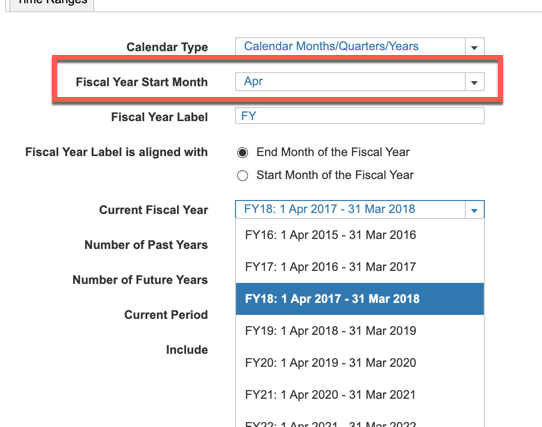# Year to Date CumulationIs there a function where I can cumulate an amount but reset it to zero when January is reached in the next year (we are using a 2 year timescale)?

Tagged:

•The following formula would work to do annual YTD cumulations where VALUE2 is the item you want to cumulate and you are using a monthly timescale:

CUMULATE('VALUE2') - OFFSET(CUMULATE('VALUE2'), -MONTH(START()), 0)

•One of our users let me know that this formula only works correctly when January is set as the fiscal year start month.  If you have a start month other than January you'll need to use this formula (which is written to work for a July start month):

CUMULATE('VALUE2') - OFFSET(CUMULATE('VALUE2'), IF MONTH(START()) > 6 THEN -MONTH(START()) + 6 ELSE -MONTH(START()) - 6, 0)

If you have a start month other than July, replace the 3 "6's" in the formula with the number below corresponding to your start month:

January - use the CUMULATE('VALUE2') - OFFSET(CUMULATE('VALUE2'), -MONTH(START()), 0) formula
February - 1
March - 2
April - 3
May - 4
June - 5
July - 6
August - 7
Sept - 8
Oct - 9
Nov - 10
Dec - 11
•If we have a week timescale, does the same logic apply?  Just replace Month function with Week Function?
•There is currently no Week function in Anaplan so what I would do here is add a new line item to hold the week number (call it "Week Number"), build an Assumption module to populate the week number and a link to pull the week number into my new line item.

Then in order to cumulate you can replace MONTH(START()) in the above formula with the new line item, "Week Number" and if using 52 week years, you would replace a 6 in the above formula with 26 (to replace # of months with number of weeks).  So the formula would look as follows:

CUMULATE('VALUE2') - OFFSET(CUMULATE('VALUE2'), IF 'Week Number' > 26 THEN -'Week Number' + 26 ELSE -'Week Number' - 26, 0)
•I think it might be an error in this formula when using other start month than January and July.

Your example works fine, because you use July (month 6 according to your table). It works because the second and the third "6" adds up to 12. If you pick another month, for example September (month 8 in your table), the third month correction should be "-4".

In the example with September, you want to offset the cumulative number from August and when standing in January, that is an offset by -5 months. We are standing in month 1 and wants to add another 4 to that subtraction.

Please correct me if I am wrong 🙂
•Seems you could get more simple construction by using PREVIOUS functions
Like
CUMULVALUE = If month(start()) = 1 then VALUE else previous(CUMULVALUE)+VALUEInstead of month(start()) = 1  trigger you could use any other time condition you need for your business case
•Thanks to Pat for coming up with this one: MOVINGSUM(VALUE, -(MONTH(START()) - 1), 0)
Note: only works with Jan start to Fiscal Year
•I find the TIMESUM formula works well for these things. Just have one line item to be the start of each year and then time sum based on that. i.e. if month(start()) = 1 then period(start()) else if month(start()) = 2 then period(start()) - 1 etc then use that as the condition in the timesum.
•Hi,

We have a start of April in our FY. I have used this formula

CUMULATE(VALUE) - OFFSET(CUMULATE(VALUE), IF MONTH(START()) > 3 THEN -MONTH(START()) + 3 ELSE -MONTH(START()) - 9, 0)

However this is summing into the next year too. Am I doing something wrong?

•This thread was before the YeartoDate function was created.  If your Fiscal Year starts in April, why not change the time settings to begin in April